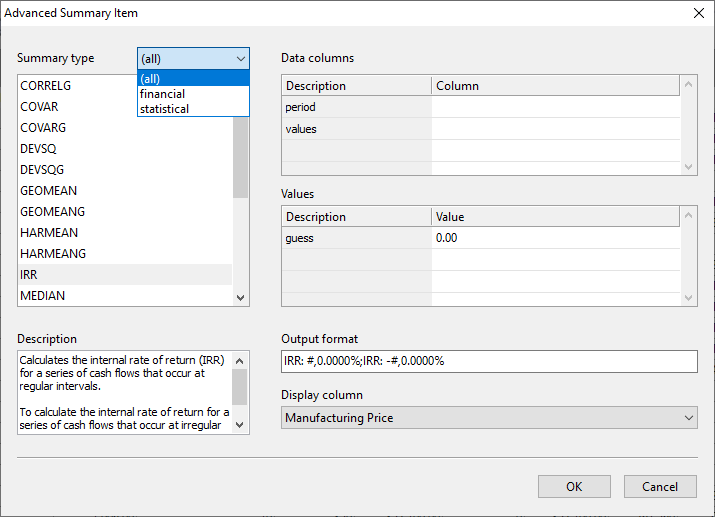Top  Previous  Next

In addition to the standard summary functions, Easy Excel Analysis can also calculate financial and statistical summaries.  To use these advanced summaries, right click on the column header and select the advanced option.The Advanced Summary Item window is displayed.  You can then select a financial or statistical function to add to your analysis table.The available statistical functions are as follows:

 AVEDEV Calculates the average of the absolute value of deviations from the mean for a given set of data. AVEDEVG Calculates the average of the absolute value of deviations from the mean for a given set of data, grouped by periods. DEVSQ Calculates the sum of the squared deviations from the mean for a given set of data. DEVSQG Calculates the sum of the squared deviations from the mean for a given set of data, grouped by periods. STDEV Calculates the standard deviation for a given set of data. STDEVG Calculate the standard deviation for a given set of data, grouped by periods. STDEVP Calculates the standard deviation for a given set of data representing an entire population. STDEVPG Calculates the standard deviation for a given set of data representing an entire population, grouped by periods. GEOMEAN Calculates the geometric mean for a set of numeric values. GEOMEANG Calculates the geometric mean for a set of numeric values, grouped by periods. HARMEAN Calculates the harmonic mean for a set of numeric values. HARMEANG Calculates the harmonic mean for a set of numeric values, grouped by periods. TRIMMEAN Calculates the mean (average) while excluding outliers. TRIMMEANG Calculates the mean (average) while excluding outliers, grouped by periods. MEDIAN Calculates the median (middle number) in a group of supplied numbers. MEDIANG Calculates the median (middle number) in a group of supplied numbers, grouped by periods. MODE Returns the most frequently occurring number in a numeric data set. CORREL Calculates the correlation coefficient between 2 numeric data sets. CORRELG Calculates the correlation coefficient between 2 numeric data sets, grouped by period COVAR Calculates the covariance, the average of the products of deviations for two data sets. COVARG Calculates the covariance, the average of the products of deviations for two data sets, grouped by periods.

The available financial functions are as follows:

 IRR Calculates the internal rate of return (IRR) for a series 'of cash flows that occur at regular intervals.   To calculate the internal rate of return for a series of cash flows that occur at irregular intervals, use the XIRR function. MIRR Calculates the modified internal rate of return (MIRR) for a series of cash flows, taking into account both discount rate and reinvestment rate for future cash flows. XIRR Calculates the internal rate of return (IRR) for a series of cash flows that occur at irregular intervals.   To calculate the internal rate of return for a series of regular, periodic cash flows, use the IRR function. NPV Calculates the net present value (NPV) of an investment using a discount rate and a series of future cash flows.   To calculate the net present value for a series of cash 'flows that occur at irregular intervals, use the XNPV function. XNPV Calculates the net present value (NPV) of an investment using a discount rate and a series of cash flows that occur at irregular intervals   To calculate the net present value for a series of regular, 'periodic cash flows, use the NPV function.